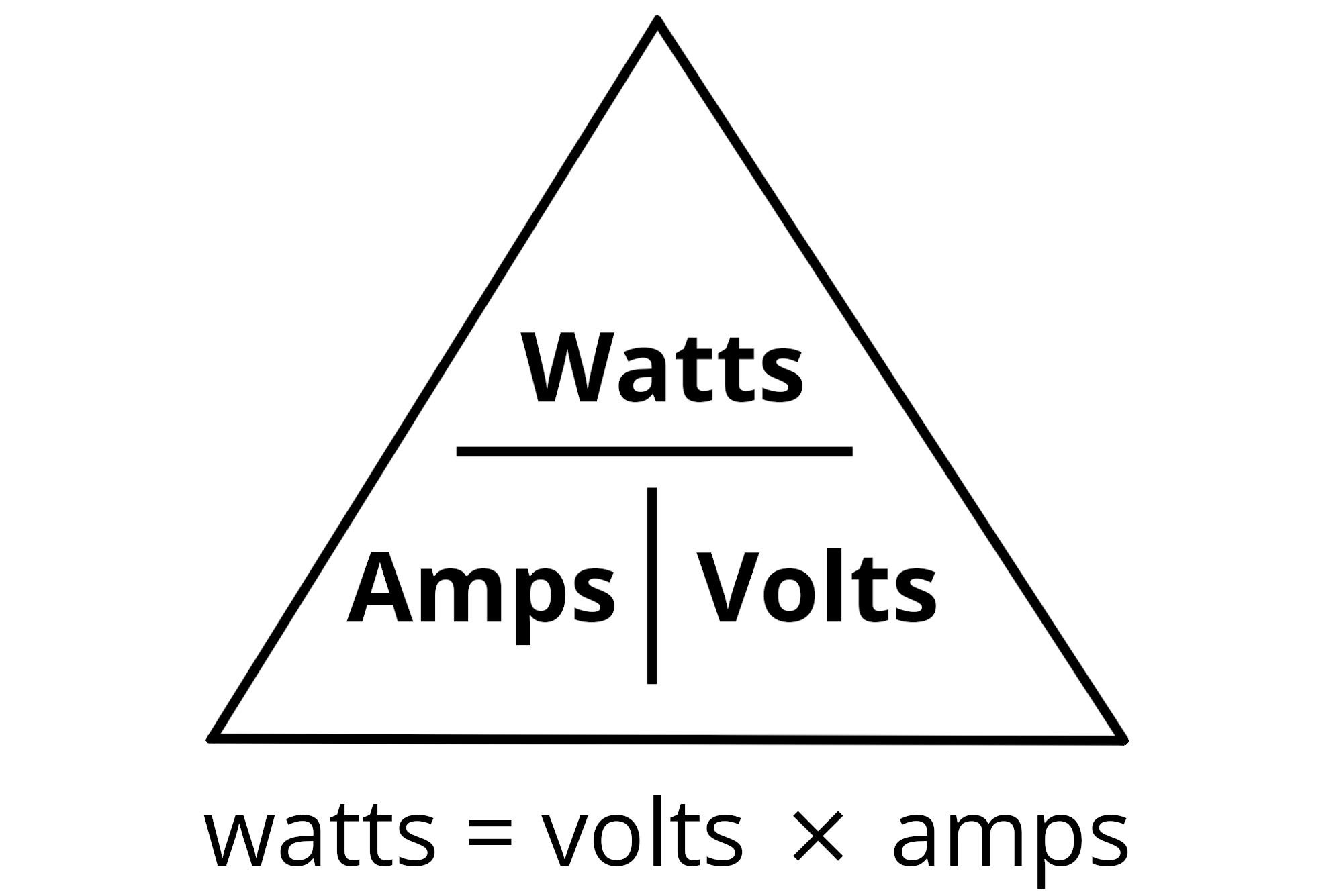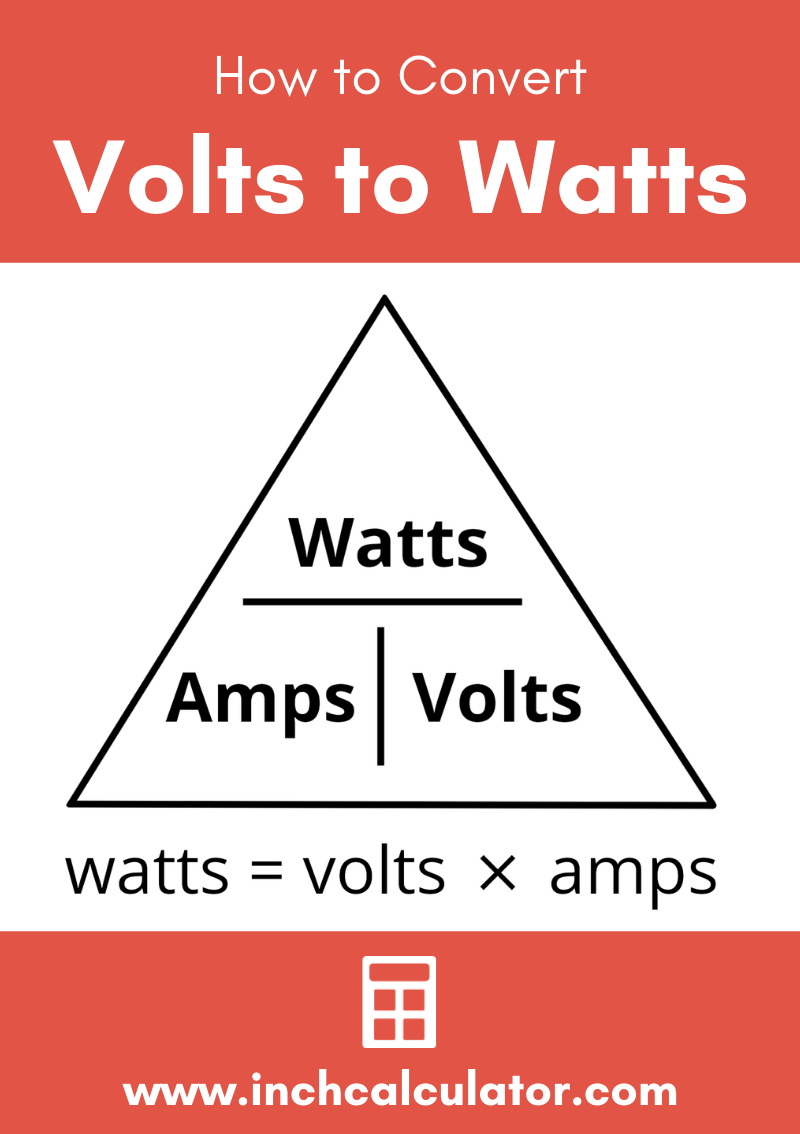# Volts to Watts Conversion Calculator

Convert volts to watts by entering the voltage and current in amps, or the resistance of the circuit in the form below.

## Power:

Watts
Learn how we calculated this below

## How to Convert Volts to Watts

Converting voltage, measured in volts, to power measured in watts is easy using the Watt’s Law power formula. Watt’s Law states that current is equal to power divided by voltage.

Multiplying both sides of this relationship by voltage, we can change this formula to also state that power is equal to voltage times current.

### Volts to Watts Formula

This is the formula to convert volts to watts, using the current in amps:

P(W) = V(V) × I(A)

Thus, the power P in watts it equal to the voltage V in volts times the current I in amps.For example, let’s convert 12 volts to watts for a DC circuit with 2 amps of current.

P(W) = 12 V × 2 A
P(W) = 24 watts

So, 12 volts will consume 24 watts of power.

## Conversion For AC Circuits

Converting voltage to wattage in AC electrical circuits uses the same formula with some slight modifications. This is because AC circuit power is comprised of a real power component, measured in watts (W), and a reactive power component, measured in volt-amps reactive (VAR).

Together, these components are called apparent power and are measured in volt-amps (VA). The real power component is the power that does the work, whereas reactive power is usually undesirable because it generates excess load on the transmission components. The ratio of real power to apparent power is called the power factor.

### Formula for AC Circuits

To account for power factor when converting volts to watts, you can use the following formula:

P(W) = V(V) × I(A) × PF

Thus, in AC circuits, power P in watts is equal to the RMS voltage V times the current I in amps times the power factor.

For example, let’s convert 120 volts to watts for an AC electrical circuit with 15 amps of current and a power factor of .9.

P(W) = 120 V × 15 A × .9
P(W) = 1,620 watts

In this example, 120 volts will consume 1,620 watts of power.

## Convert Volts to Watts using Resistance

It’s also possible to convert volts to watts for a simple resistive load if you know the resistance of the circuit. Use this formula to convert volts to watts using the resistance.

P(W) = V(V)2 ÷ R(Ω)

Thus, the power P in watts is equal to voltage V squared, divided by the resistance R in ohms.

For example, let’s convert 24 volts to watts for a DC circuit with 12 ohms of resistance.

P(W) = (24 V)2 ÷ 12 Ω
P(W) = 576 V ÷ 12 Ω
P(W) = 48 watts

The result is 48 watts.

## Equivalent Volts and Watts Measurements

Equivalent volts and watts at various current ratings
Voltage Power Current
1 volt 1 watt 1 amp
1 volt 2 watts 2 amps
1 volt 3 watts 3 amps
1 volt 4 watts 4 amps
2 volts 2 watts 1 amp
2 volts 4 watts 2 amps
2 volts 6 watts 3 amps
2 volts 8 watts 4 amps
3 volts 3 watts 1 amp
3 volts 6 watts 2 amps
3 volts 9 watts 3 amps
3 volts 12 watts 4 amps
4 volts 4 watts 1 amp
4 volts 8 watts 2 amps
4 volts 12 watts 3 amps
4 volts 16 watts 4 amps
5 volts 5 watts 1 amp
5 volts 10 watts 2 amps
5 volts 15 watts 3 amps
5 volts 20 watts 4 amps
6 volts 6 watts 1 amp
6 volts 12 watts 2 amps
6 volts 18 watts 3 amps
6 volts 24 watts 4 amps
7 volts 7 watts 1 amp
7 volts 14 watts 2 amps
7 volts 21 watt 3 amps
7 volts 28 watts 4 amps
8 volts 8 watts 1 amp
8 volts 16 watts 2 amps
8 volts 24 watts 3 amps
8 volts 32 watts 4 amps
9 volts 9 watts 1 amp
9 volts 18 watts 2 amps
9 volts 27 watts 3 amps
9 volts 36 watts 4 amps
10 volts 10 watts 1 amp
10 volts 20 watts 2 amps
10 volts 30 watts 3 amps
10 volts 40 watts 4 amps
11 volt 11 watt 1 amp
11 volt 22 watts 2 amps
11 volt 33 watts 3 amps
11 volt 44 watts 4 amps
12 volts 12 watts 1 amp
12 volts 24 watts 2 amps
12 volts 36 watts 3 amps
12 volts 48 watts 4 amps
24 volts 24 watts 1 amp
24 volts 48 watts 2 amps
24 volts 72 watts 3 amps
24 volts 96 watts 4 amps
48 volts 48 watts 1 amp
48 volts 96 watts 2 amps
48 volts 144 watts 3 amps
48 volts 192 watts 4 amps
120 volts 120 watts 1 amp
120 volts 240 watts 2 amps
120 volts 360 watts 3 amps
120 volts 480 watts 4 amps
240 volts 240 watts 1 amp
240 volts 480 watts 2 amps
240 volts 720 watts 3 amps
240 volts 960 watts 4 amps

Learn more Ohm’s Law electrical formulas and see more conversions on our Ohm’s Law calculator.## References

1. Miller, C., Ugly’s Electrical References, 2020 Edition, 2020, Jones & Bartlett Learning, 16-23. https://books.google.com/books?id=1kS8DwAAQBAJ